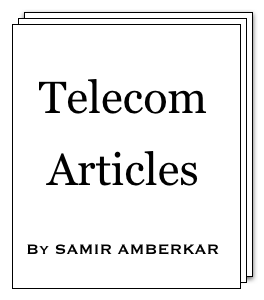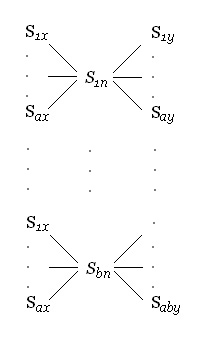online LTE test online C test Updated or New CDF explained5G Page5G Intent (a presentation)5G Intent (article)CV2X PageA Look at CV2X (a presentation)

 About Feedback Information Theory Modulation Multiple Access OSI Model Data Link layer SS7 Word about ATM GSM GPRS UMTS WiMAX LTE 5G Standard Reference Reference books Resources on Web Miscellaneous CV2X Mind Map Perl resources Magic MSC tool Bar graph tool C programming ASCII table Project Management Simple Google box HTML characters Site view counter 7 5 0 , 5 4 0 (May-2010 till Mar-2019)Rate in terms of noise entropy

Rate in terms of noise entropy [Under Information theory > Shannon's paper]

Let us put down our findings from article on Information rate with noise and try to combine them.

1) R = H(x)Hy(x)

2) H(x,y)= H(x) + Hy(x)

3) H(x,n) = H(x) + H(n)

Here destination (y) symbols are produced by impact of noise (n) symbols on source (x) symbols. This mean each y symbol correspond to combination of certain x symbol and n symbol. So number of combinations of x and y are same as number of combinations of x and n. In other words, average number of bits per symbol required to represent combined x and n signals is same as average number of bits per symbols required to represent combined x and y signals.

That is: 4) H(x,y)= H(x,n)

Combining above equations, we get R = H(y)H(n). This theorem 16 (page 43) from Shannon's paper.

Can we directly try to come out with above equation ? Consider following diagram:Each Sx is combined with each Sn (based on probabilities) to give us Sy. As x and n are independent, whatever are the individual probabilities (uncertainities or entropies) of x and n, the diagram hold true. If n is not present or it has zero entropy (uncertainty), each y symbol will map to each x symbol. Due to presence of n's symbols (non-zero entropy), more number of bits are needed for y as shown in above diagram. If you examine the diagram and try b = 1,2,4,...., it will be evident that to get actual bits per symbol, we must deduct noise introduced n's bits per symbol from y's observed bits per symbol.

That is: R = H(y)H(n)